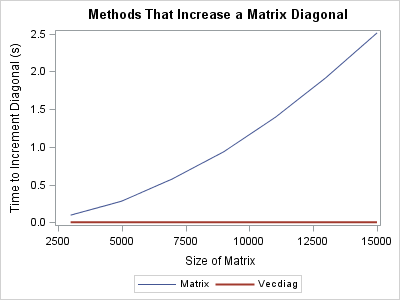I was recently asked about how to use the SAS/IML language to efficiently add a constant to every element of a matrix diagonal. Mathematically, the task is to form the matrix sum A + kI, where A is an n x n matrix, k is a scalar value, and I is the identity matrix. The person who asked the question was dealing with large matrices. He knew that explicitly forming the identity matrix is inefficient and that adding n2n zeros to A is unnecessary, but he wasn't sure how to avoid it.

I have previously blogged about how to assign the diagonal elements of a matrix. In that post I wrote a little helper function that will set the diagonal of a matrix to a given vector or scalar value. The function is repeated below:

```proc iml; start SetDiag(A, v); diagIdx = do(1,nrow(A)*ncol(A), ncol(A)+1); /* index diagonal elements */ A[diagIdx] = v; /* set diagonal elements */ finish;```

This function sets the diagonal elements of the matrix A to the value v, which can be a vector or a scalar. To increment (rather than replace) the diagonal, use the VECDIAG function to extract the current diagonal values of the matrix. Add a constant and call the function like this:

```m = j(5000, 5000, 1); /* create a 5000 x 5000 matrix */ run SetDiag(m, vecdiag(m) + 100); /* increment diagonal elements by 100 */```

### Timing the performanceRecall that it is easy to use the TIME function in SAS to compare the performance of two competing algorithms. The plot at the left shows the time required to increment a matrix diagonal for a sequence of large matrices, up to 15,000 x 15,000. The blue line represents the naive computation
A + kI. Notice that the time increases quadratically with the size of the matrix because this algorithm adds n2 numbers to the matrix.

In contrast, the red line shows the time that it takes to form a vector that indexes the diagonal elements of a matrix and to replace only those elements. Theoretically, the time should be linear with the size of the matrix, but the computation is so fast that the elapsed time registers as instantaneous for even the largest matrix.

In summary, when working with large matrices, you can save lots of computational effort if you avoid unnecessary computations. Never multiply or add large diagonal matrices unless absolutely necessary. Instead, think about whether it is possible to index into the matrix and change only those numbers that you need to change.

By the way, increasing the diagonal of a matrix is sometimes called "ridging a matrix" and it is used in ridge regression. Ridging a matrix is also sometimes used in numerical computations that involve estimated covariance matrices. Sometimes an estimate is not positive definite, and ridging is one approach to try to obtain an estimate for a covariance matrix that has this important property.

Share